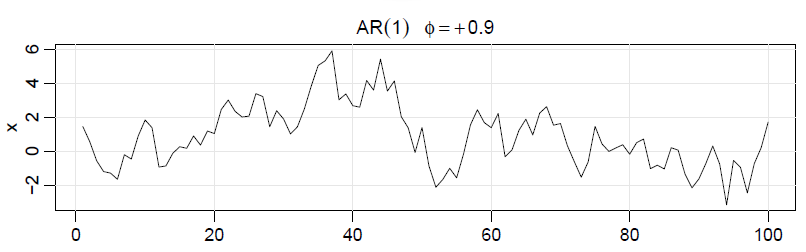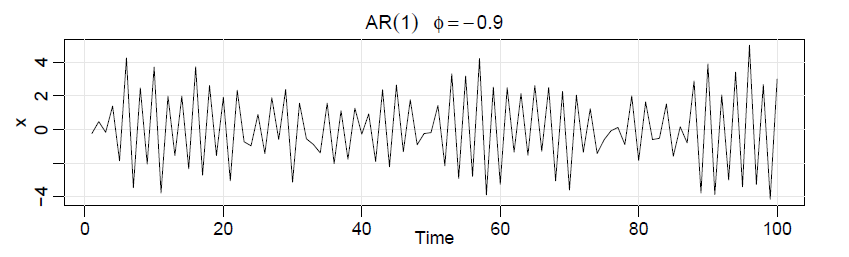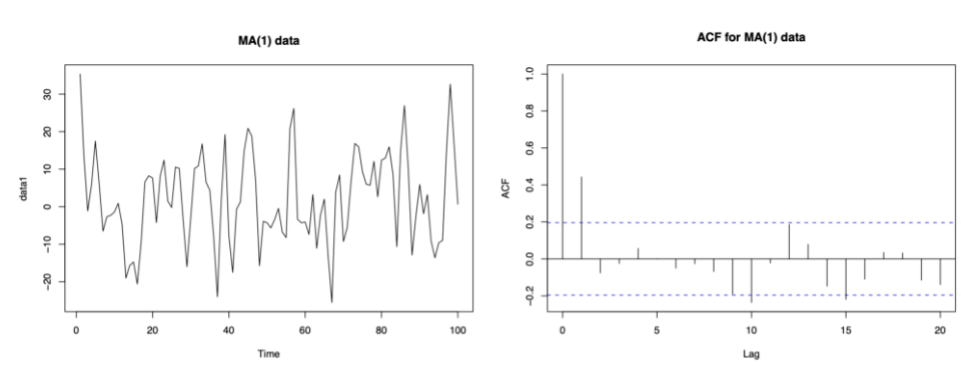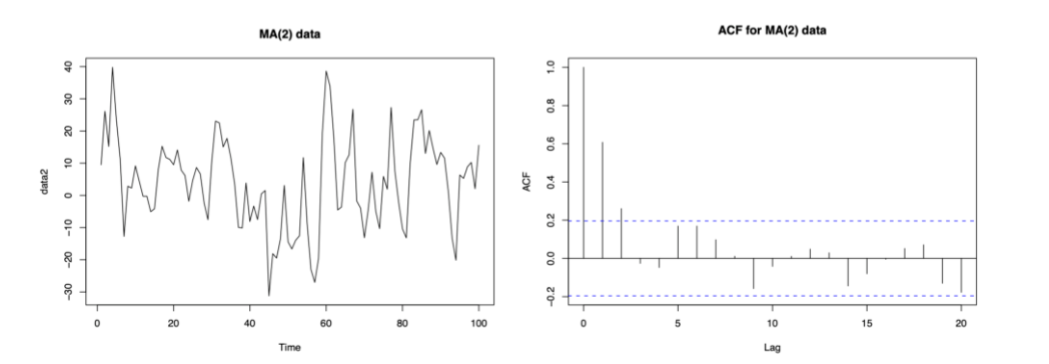# AR, MA, and ARIMA Models: A Comprehensive Guide

In the first part of this series, I have discussed the following questions.

• What is Time Series?
• What is the main focus of Time Series?
• How is Time Series different from Regression?
• How to mathematically model Time Series?
• Why stationarity of the Time Series?
• What is the Central Idea (the two fundamental steps)?
• What is ARIMA modeling in short?

# Step 1

`Exploratory Data Analysis and Transform data into stationary data.`

# Step 2

`Model and Predict the dependence structure of the errors.`

## In this article, I will discuss Step 2, using AR, MA, and ARIMA.

I will discuss the following questions:

• What is stationary data?
• What are the components behind prediction?
• How future data depend on the past errors made?
• What is MA(q)?
• What is AR(p)?
• How to get a good estimate of q and the coefficients in MA(q)?
• Why do we need PACF?
• How to get a good estimate of p and the coefficients in AR(p)?
• What is ARMA(p,q)?
• How to estimate the parameters of ARMA(p,q)?
• What is Ljung Box Test?
• What is ARIMA(d,p,q)?
• How to estimate the parameters of ARIMA(d,p,q)?

# What is Stationary Data?

After step 1, we get stationary data. Let’s say Y is a “stationarized” data

A “stationarized” time series has

• no trend,
• a constant variance over time, and
• constant “wiggliness” over time, i.e., its random variations have a qualitatively similar pattern at all points in time if you squint at the graph.

In technical terms, this means that its autocorrelations are constant over time.

At this point, we have stationary data (say zero mean), with no seasonality.

We also have the white noise in the prediction, the uncorrelated errors.

# What are the components behind prediction?

Understand that prediction needs to take care of the following.

• Future Data
• To how much extent the future data is dependent on the past data?
• To how much extent the past predictions are doing well and correspondingly change the prediction style?

# How future data depend on the past errors made?

Let’s say, you have observed that in the past 2 days, you have observed a consistent error in prediction around negative 20%, so in the next day, you must also predict not the actual predicted value, but the actual predicted value with a reduction in 20% following the behavior of the errors.

Hence, we come to the Moving Average method.

# What is MA(q) (Moving Average)?

In moving average, we check how the stationary time series is dependent on the errors, in an additive way.

q: denotes the number of past errors the future is dependent upon.

We will come to the ideas of how to estimate this q and the thetas from data.

# What is AR(p) (Autoregressive)?

In autoregressive, we check how the stationary time series is dependent on the past data, in an additive way. This is exactly like the multivariate regression step, hence the name autoregressive.

p: denotes the number of past data the future is dependent upon.

We will come to the ideas of how to estimate this p and the thetas from data.See how the positive effect on just the previous data results in a continuation of similar paths.See how the negative effect on just the previous data results in a change of signs so often.

# How to get a good estimate of q and the coefficients in MA(q)?

If you calculate the ACF function of MA(q), it will be 0 after time lag = q.

The cutting off of ACF(h) after q lags is the MA's signature (q) model.

ExamplesObserve that the ACF lies outside the two confidence interval bars only till MA(1).Observe that the ACF lies outside the two confidence interval bars only till 2. Hence MA(2)

The coefficient estimation is done by

• Ordinary Least Square
• Fast Filtering Algorithm, etc

# Why do we need the Partial Autocorrelation Function (PACF)?

If we try to get a good estimate of p of the AR(p) model, the ACF doesn’t give any insight, because it behaves similarly.

So, we need another tool, which can capture the relationship between the future data and the past data points.

## What is PACF (Partial Autocorrelation Function)?

In general, a partial correlation is a conditional correlation. It is the correlation between two variables under the assumption that we know and takes into account the values of some other set of variables.

For a time series, the partial autocorrelation of lag h, between the data point at t, and time t-h, is defined as the conditional correlation between the data point at t, and time t-h, conditional on the set of observations that come between the time points t and t−h.

2nd Order Lag PACF

3rd Order Lag PACF

For an AR model, the theoretical PACF “shuts off” past the order of the model.

`Exercise: Think, why PACF gives a better idea than ACF?`

# How to get a good estimate of p and the coefficients in AR(p)?

If you calculate the PACF function of AR(p), it will be 0 after time lag = p.

The cutting off of PACF(h) after p lags is the AR’s signature (p) model.

Examples

The coefficient estimation is done by

• Ordinary Least Square
• transient Kalman gain, etc

# What is ARMA(p,q)?

When the AR(p) and the MA(q) models are combined together to give a general model, we call it ARMA (p,q) to model stationary nonseasonal time series data.

We need to estimate the parameters of ARMA (p,q) now.

# How to estimate the parameters of ARMA(p,q)?

We have understood the parameters p, q by observing the ACF and PACF plot.

But, we will discuss a general algorithm now.

This is a model selection problem. We minimize the BIC (Bayesian Akaike Criterion) and select among all the models, the one with minimum BIC.

In order to determine which order p,q of the ARMA model is appropriate for a series, we need to use the AIC (or BIC) across a subset of values for p,q.

But, now after the fitted model with ARMA, how do we know that the errors are not again autocorrelated?

# What is Ljung Box Test?

The Ljung-Box test is a classical hypothesis test that is designed to test whether a set of autocorrelations of a fitted time series model differ significantly from zero.

The test does not test each individual lag for randomness but rather tests the randomness over a group of lags.

We define the null hypothesis H0 as The time-series data at each lag are iid that is, the correlations between the population series values are zero.

We define the alternate hypothesis Ha as The time series data are not i.i.d. and possess serial correlation.

So, after fitting the ARMA(p,q) model, we must apply the Ljung-Box test to determine if a good fit has been achieved, for particular values of p,q.

# ARIMA = AR + I + MA = I + ARMA

ARIMA is actually to model a time series with a trend added with stationary errors.

## Step 1

By differencing in I step, first we detrend the time series to get the stationary time series errors.

## Step 2

Then, we apply ARMA modeling to this remaining portion.

Simple and Elegant.

I will next share the practical approach to do Time Series Analysis which will include Step 1 and the fitting of the ARIMA model.

Stay Tuned. Stay Blessed.

Don’t forget to clap and follow, if you have enjoyed reading this.

--

--